# Fractions - 9th grade (14y) - math problems

#### Number of problems found: 205

• Mrs PerryMrs Perry shares out 25 biscuits between Gemma and Zak in the ratio 1:4. How many biscuits has each?
• Earning 2Rahul earns 5000 Rs, he saves 500 Rs . What's percentage of his earnings does he save? *
• Garden GThe rectangular, trapezoidal garden has a base length of 81m, 76m, and a vertical arm of 12m. Calculate how many m2 of the area will remain for planting greenery if 1/3 of the area is built. Calculate the consumption of mesh for land fencing.
• Two prizes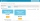In a class of 15 pupils (7 boys and 8 girls), Teacher Mae is giving away a random prize. What is probability that both prizes are won by girls?
• Proportional relationship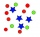The ordered pairs (6,24) and (1, s) represent a proportional relationship. Find the value of s.
• One dice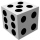Calculate the probability of one dice roll with the numbers 1, 2, 3, 4, 5, 6 on the walls. Write the results in a notebook in the shape of a fraction in the basic form: 2/3. a, The number 1 falls on the cube. b, The number 5 falls on the cube. c, An even
• Secondary school1/2 of the pupils want to study at the secondary school, 1/4 at the apprentice, 1/6 at the grammar school three pupils do not like to learn. How many students are in the class?
• Drawing from a hatWhen drawing numbers from a hat from 1 to 35, we select random given numbers. What is the probability that the drawn numbers will be divisible by 8 and 2?
• Say we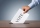Say we have 70 voting delegates and we need a 2/3 vote What would that number be? Also same as above but in this virtual world, it converts to percentages. What would the % number of positive votes be?
• Equilateral coneWe pour so much water into a container that has the shape of an equilateral cone, the base of which has a radius r = 6 cm, that one-third of the volume of the cone is filled. How high will the water reach if we turn the cone upside down?
• Final exam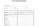At the final exam, the student answers from three areas, which are evaluated in a ratio of 1: 2: 2. What grade will John receive if he answered as follows: 3,1,2.
• A farmer's heritage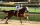A farmer died leaving his 17 horses to his 3 sons. When his sons opened up the Will it read: My eldest son should get 1/2 (half) of total horses; My middle son should be given 1/3rd (one-third) of the total horses; My youngest son should be given 1/9th (o
• Double ratio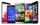The mobile phone was twice gradually discounted in the ratio of 3: 2 1 half: 5 quarters. How much did it originally cost if the price was CZK 4,200 after a double discount?
• The number 72The number 72 increase by 25%. By how much % will you have to reduce the number you created to get the number 72 again?Adam has half the money in his right pocket than in his left pocket. If he transferred 40 crowns from the left pocket to the right, he would have the same in both pockets. Calculate how many crowns does Adam has in his left pocket more than in his right?
• Half of halvesHalf of the square we cut off, then half of the rest, etc. Five cuts we made in this way. What part of the content of the original square is the content of the cut part?
• The angles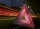The angles in the triangle are in the ratio 12: 15: 9. Find the angles.
• Cllub flag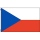The children had the club flag sewn on. The red color covered 2/20 of the total area of the flag, the blue area a fifth, the yellow 19% of the total area. What% is the green area occupied?
• The fourThe four pirates divided 65 coins to each other. They were sorted by age, the youngest receiving the least number of coins, each half more than the previous one. How many coins did the oldest pirate receive?
• The dealer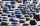The dealer planned to sell 40% of the cars he had in stock in May. However, he sold 20 less cars, ie only 15% of cars. a) How many cars did he have in stock? b) How many cars did he sell in May?

Do you have an exciting math question or word problem that you can't solve? Ask a question or post a math problem, and we can try to solve it.

We will send a solution to your e-mail address. Solved examples are also published here. Please enter the e-mail correctly and check whether you don't have a full mailbox.

Need help to calculate sum, simplify or multiply fractions? Try our fraction calculator. Fraction Word Problems. Examples for 9th grade.